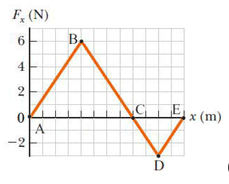Question
12 views

The force acting on a particle varies as in the figure below. (The x axis is marked in increments of 1.50 m.)

Find the work done by the force as the particle moves across the following distances.

(a) from x = 0 m to x = 12.0 m

(b) from x = 12.0 m to x = 18.0 m

(c) from x = 0 m to x = 18.0 mhelp_outlineImage TranscriptioncloseF, (N) B. 6. 4 NG x (m) -2 fullscreen
check_circle

Step 1

Work done = area under graph

Step 2

a) From 0-12

Work done

Step 3

b) From 12-18

Work done

...

### Want to see the full answer?

See Solution

#### Want to see this answer and more?

Solutions are written by subject experts who are available 24/7. Questions are typically answered within 1 hour.*

See Solution
*Response times may vary by subject and question.
Tagged in

### Work,Power and Energy